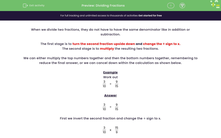# Divide Fractions

In this worksheet, students will divide two simple fractions.Key stage:  KS 3

Curriculum topic:   Number

Curriculum subtopic:   Use Four Operations for All Numbers

Difficulty level:#### Worksheet Overview

In this activity, we will practise dividing fractions.

The method we're going to use is KFCNo, not the takeaway chicken - something much better!

KFC stands for Keep Flip Change

First, we keep the first number or fraction unchanged.

Next, we flip the second fraction upside down.

Finally, we change the sign between the two fractions or numbers from ÷ to x.

So, 3/4 ÷ 2/5 becomes 3/4 x 5/2

Having done that, we can multiply the top numbers together and then the bottom numbers together, remembering to reduce the final answer. Sometimes, we can cancel down within the calculation before doing any multiplying, as shown below.

Example

Work out

 3 ÷ 9 10 15

First, we keep the first fraction unchanged.

Next, we flip the second fraction.

Finally, we change the ÷ sign to x.

 3 x 15 10 9

We could just multiply the top numbers together: 3 x 15 = 45 and the bottom numbers 10 x 9 = 90

This would give us 45/90 which cancels down to 1/2.

However, it is also possible to cancel down before doing the calculation!

Look for any top number that has a common factor with any bottom number.

If we find such a reducible pair of numbers, we divide them by their common factor.

 3 x 15 10 9

 = 3 ÷ 3 x 15 10 9 ÷ 3

 = 1 x 15 10 3

 = 1 x 15 ÷ 5 10 ÷ 5 3

 = 1 x 3 2 3

 = 1 x 3 ÷ 3 2 3 ÷ 3

 = 1 x 1 2 1

 = 1 2

Let's get started!### What is EdPlace?

We're your National Curriculum aligned online education content provider helping each child succeed in English, maths and science from year 1 to GCSE. With an EdPlace account you’ll be able to track and measure progress, helping each child achieve their best. We build confidence and attainment by personalising each child’s learning at a level that suits them.

Get started